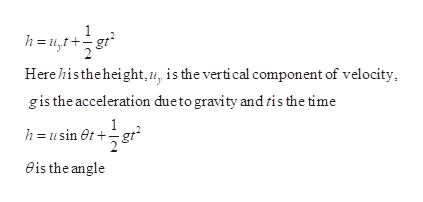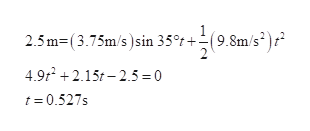# A boy throws a baseball onto a roof and it rolls back down andoff the roof with a speed of 3.75 m/s. If the roof is pitched at35.0° below the horizon and the roof edge is 2.50 m above theground, find (a) the time the baseball spends in the air, and(b) the horizontal distance from the roof edge to the pointwhere the baseball lands on the ground.

Question
2 views

A boy throws a baseball onto a roof and it rolls back down and
off the roof with a speed of 3.75 m/s. If the roof is pitched at
35.0° below the horizon and the roof edge is 2.50 m above the
ground, find (a) the time the baseball spends in the air, and
(b) the horizontal distance from the roof edge to the point
where the baseball lands on the ground.

check_circle

Step 1

a)

The total time baseball spends in air can be determined using the equation of motionhelp_outlineImage Transcriptioncloseh = u,t+ Here histheheight,u, is the vertical component of velocity, gis the acceleration dueto gravity and tis the time h = u sin et + gt eis the angle fullscreen
Step 2

Then,help_outlineImage Transcriptionclose2.5 m=(3.75m/s )sin 35°t +(9.8m/s )t 4.9t + 2.15t – 2.5 = 0 t= 0.527s fullscreen
Step 3

b)

Horizontal distance ...

### Want to see the full answer?

See Solution

#### Want to see this answer and more?

Solutions are written by subject experts who are available 24/7. Questions are typically answered within 1 hour.*

See Solution
*Response times may vary by subject and question.
Tagged in

### Kinematics